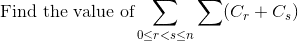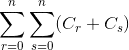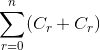# Binomial Series

zorro

## Homework Statement## The Attempt at a Solution

Is there any difference between the above expression and?

Is there any relation between these two?

Homework Helper

## Homework StatementAre you sure there are up to 2 sigma signs in that expression? By the way, you mean $$C_r^n$$ right?

If there's just one sigma, then $$\sum_{0 \le r < s \le n} (C_r^n + C_s^n)$$ is different from $$\sum_{r = 0}^n \sum_{s = 0}^n (C_r^n + C_s^n)$$.

In the first sum $$\sum_{0 \le r < s \le n} (C_r^n + C_s^n)$$, r, and s can take any value raging from 0 to n, but r must be less than s.

However, in the second sum: $$\sum_{r = 0}^n \sum_{s = 0}^n (C_r^n + C_s^n)$$, r, and s can take any value raging from 0 to n, no more requirement is needed.

So, in general, the second sum will have more terms than the first sum.

Homework Helper
Hi Abdul!The second one is roughly double the first, since it contains eg C1 + C2 but not C2 + C1.

hmm … what about all the terms such as C1 + C1 ?can you find an exact equation for the difference between the second and twice the first?zorro
Are you sure there are up to 2 sigma signs in that expression?

Yeah there are 2 sigma signs. 0<=r<s<=n is in between the two sigma signs.

can you find an exact equation for the difference between the second and twice the first?

Does that equate to?

Homework Helper
Yes, except i'd call it 2 ∑Crok now write ∑∑ (Cr + Cs) over all r and s in terms of ∑Cr(try it first with an easy small number for n, like n = 3, if you're stuck)

zorro
Thanks!.... I got the answer